Saturday, August 15, 2020
Home > geometry > SSC CGL Geometry Shaded Area Set 2

# SSC CGL Geometry Shaded Area Set 2

SSC CGL CHSL Shared Area & Perimeter
Shaded Area & Perimeter Questions Sets
Set 1Set 2Set 3

SSC CGL Geometry Shaded Area Set 2 : Ques No 1

In the figure below, a semi-circle and two quarter circles are drawn in a square of side 10 units, find the shaded area.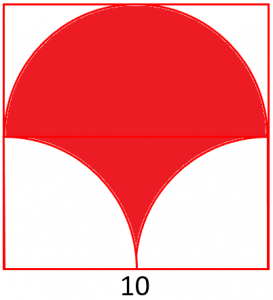Options:

A.

B.

C.

D.

Solution:

SSC CGL Geometry Shaded Area Set 2 : Ques No 2

In the figure below, two right triangles and one quarter circle are drawn in a square of side 4 units, find the shaded area.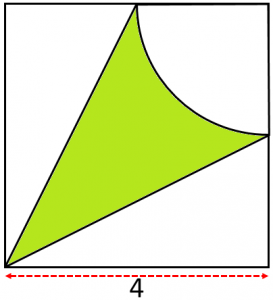Options:

A.

B.

C.

D.

Solution:

SSC CGL Geometry Shaded Area Set 2 : Ques No 3

In the figure below, a square is of side x units, find the shaded area in terms of x.Options:

A.

B.

C.

D.

Solution:

SSC CGL Geometry Shaded Area Set 2 : Ques No 4

In the figure below, two concentric circles are drawn with radii a units and 2a units, find the shaded area in terms of a.Options:

A.

B.

C.

D.

Solution:

SSC CGL Geometry Shaded Area Set 2 : Ques No 5

In the figure below, two concentric circles are drawn with radii 1 units and 2 units in a square, find the shaded area.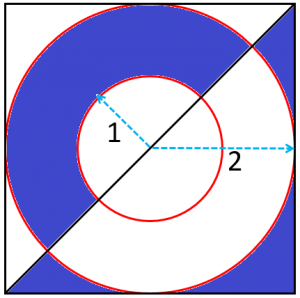Options:

A.

B.

C.

D.

Solution:

SSC CGL Geometry Shaded Area Set 2 : Ques No 6

In the figure below, two circles of equal radii 2 units touch each other externally and two direct common tangents are drawn, find the shaded area.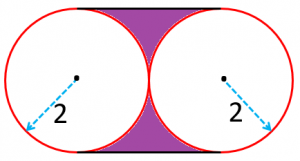Options:

A.

B.

C.

D.

Solution:SSC CGL Geometry Shaded Area Set 2 : Ques No 7

In the figure below, ABCD is a square of side 12 units, find the shaded area.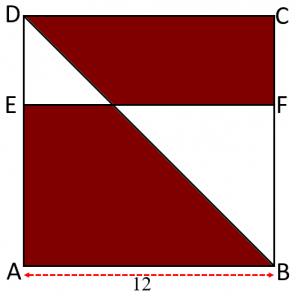Options:

A.

B.

C.

D.

Solution:

SSC CGL Geometry Shaded Area Set 2 : Ques No 8

In the figure below, a square is of side 10 units, find the shaded area.Options:

A.

B.

C.

D.

Solution:

SSC CGL Geometry Shaded Area Set 2 : Ques No 9

In the figure below, an equilateral triangle is of side 6 units, find the shaded area.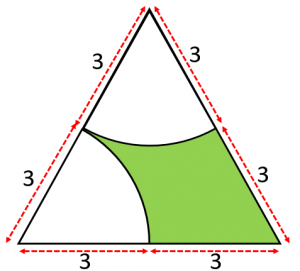Options:

A.

B.

C.

D.

Solution:

SSC CGL Geometry Shaded Area Set 2 : Ques No 10

In the figure below, a square is of side 10 units, find the shaded area.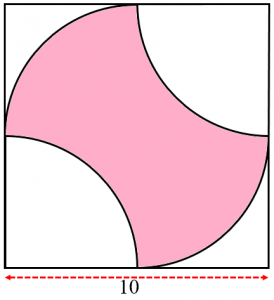Options:

A.

B.

C.

D.

Solution:

SSC CGL Geometry Shaded Area Set 2 : Ques No 11

What fraction of this square region is shaded? Stripes are equal in width, and the figure is drawn to scale?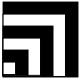Options:

A. 5/12

B. 1/2

C. 7/12

D. 2/3

E. 5/6

SSC CGL Geometry Shaded Area Set 2 : Ques No 12

Each side of the large square in the figure is trisected (divided into three equal parts). The corners of an inscribed square are at these trisection points, as shown. The ratio of the area of the inscribed square to the area of the large square isOptions:

A. 5/12

B. 1/2

C. 7/12

D. 2/3

E. 5/6

SSC CGL Geometry Shaded Area Set 2 : Ques No 13

Dots are spaced one unit apart, horizontally and vertically. The number of square units
enclosed by the polygon is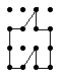Options:

A. 5

B. 6

C. 7

D. 8

E. 9

SSC CGL Geometry Shaded Area Set 2 : Ques No 14

What is the ratio of the area of the shaded square to the area of the large square? (The figure is drawn to scale.)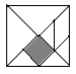Options:

A. 1/6

B. 1/7

C. 1/8

D. 1/12

E. 1/16